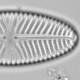## Finding singletons in real data

I have argued that the rarest species in an assemblage should typically be represented by a single individual, and that this can be used to estimate the count sum when this is not reported.

Now I want to test this with some real data. Starting with tree-count data from Barro Colorado Island which is available from within the `vegan` package. How many species in each of the 50 samples are represented by a single individual?

```library("tidyverse")
data("BCI", package = "vegan")

data_frame(n = rowSums(BCI == 1)) %>%
ggplot(aes(x = n)) +
geom_bar(width = 1) +
labs(x = "Number of singletons") +
xlim(21, NA)
```Number of singletons per sample in the BCI data-set

All of the BCI samples have many singletons: the probability of finding a sample without any singletons is obviously very small. But perhaps some attributes of the relatively large (median count is 428 trees) species rich (median richness is 91 species) samples are not relevant to chironomid or diatom samples.

What happens when the count sum is much smaller? Here, I resample with replacement each BCI sample to a count of fifty trees. This is done one thousand times.

```BCI_50 <- BCI %>%
rowwise() %>%
do(
as_data_frame(
t(rmultinom(n = 1000, size = 50, prob = .))
)
)

n_50 <- data_frame(n = rowSums(BCI_50 == 1)) n_50 %>%
ggplot(aes(x = n)) +
geom_bar(width = 1) +
labs(x = "Number of singletons")
```Number of singletons per sample in the BCI data-set when the count sum is reduced to 50 trees.

The minimumum number of singletons in the resampled data is now 3, and 99.97% of samples have more that 5 singletons. Even with a count of only fifty, samples without singletons are going to be rare.

How about if the taxonomic resolution is reduced by reanalysing the data by genus rather than species? That turns out not to change things much as many genera have only one species in each sample. The richness can be greatly reduced by grouping species according to the first letter of the genus name.

```BCI_50_genera <- BCI_50 %>%
rowid_to_column("sample") %>%
gather(key = species, value = count, -sample) %>%
# mutate(genera = gsub("^(\\w+).*", "\\1", species)) %>%
mutate(genera = gsub("^(\\w{1}).*", "\\1", species)) %>%
group_by(sample, genera) %>%
summarise(count = sum(count))

n_50_genera <- BCI_50_genera %>%
summarise(n = sum(count == 1))

n_50_genera %>%
ggplot(aes(x = n)) +
geom_bar(width = 1) +
labs(x = "Number of singletons")
```Number of singletons per sample in the BCI data-set when the count sum is reduced to 50 trees and the taxonomic resolution is greatly reduced.

The mean richness is now 15.2 taxa.
99.53% of samples have at least one singleton amongst the 50 trees. Samples without singletons are still rare.

Time to move over to some chironomid data. Professor Ian Walker archived chironomid stratigraphies from nine Canadian lakes. The data are in tilia files (not recommended), fortunately the `rioja` package has a function to read tilia files. Unfortunately, some characters are not being read correctly and are crashing `read.Tilia` so I am calling the underlying C code directly and removing taxa after ‘unidentifiable’, which removes all the other diptera and sums that I don’t need, as well as all the problem characters.

```library("rioja")
obj <- .Call("ReadTiliaFile", fname, PACKAGE = "rioja") obj\$Data %>%
set_names(make.names(obj\$FullNames, unique = TRUE)) %>%
as_data_frame() %>%
rowid_to_column(var = "sample") %>%
select(1:grep("^UNIDENTIFIABLE\$", names(.), ignore.case = TRUE))
}

tilia_files <- dir(pattern = "\\.til\$", recursive = TRUE, full.names = TRUE, ignore.case = TRUE)

tilia_chironomids <- tilia_files %>%
set_names(gsub(pattern = ".*/(\\w*)\\.til\$", replacement = "\\1", x = ., ignore.case = TRUE)) %>%

min_counts <- tilia_chironomids %>%
filter(lake != "brier") %>% #percent data
gather(key = species, value = count, -lake, -sample) %>%
mutate(count = round(count * 2) / 2 ) %>% # Wood Lake counts back-transformed from % data
filter(count > 0) %>%
group_by(lake, sample) %>%
summarise(min_count = min(count), sum_count = sum(count), n_sing = sum(count <= 1), nsp = n())
```

The chironomids are mainly identified to genus level, some higher, some lower. Of the 158 (median count sum = 86.25, median richness = 18), 98.1% have singletons (whole or half). The median number of singletons is 6.

Of the three samples without singletons, one has a count sum of only 4.5. The other two have sensible looking counts: not all the counts are multiples of the lowest count, which would help recognise this case.

I am confident that assemblages will generally have one or more singletons, so they can be used to estimate the count sum when this is not given. This analysis shows that samples with low diversity (or equivalently low taxonomic resolution), especially with large counts, or very small counts sums might not. Analyst-related issues that increase the risk of singletons being absent include counting only a limited range of taxa (as is sometimes done in pollen analysis) and archiving the data after rare taxa have been deleted (please archive the full data).